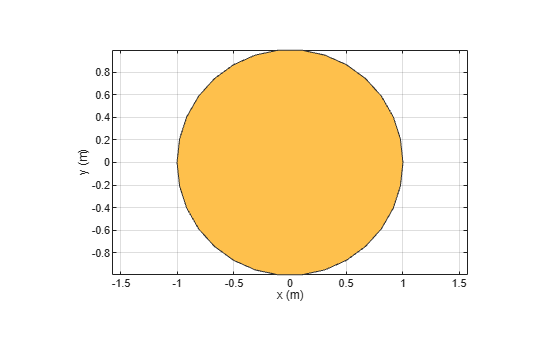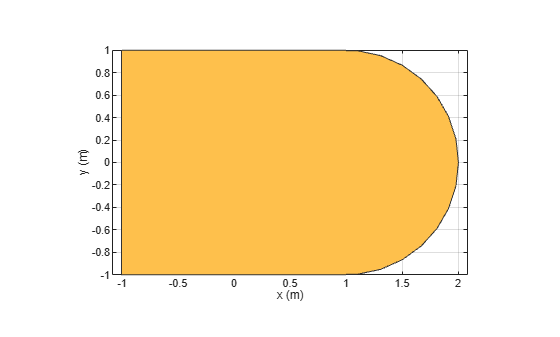# antenna.Circle

Create circle centered at origin on xy-plane

## Description

Use the `antenna.Circle` object to create a circle centered at the origin and on the xy-plane. You can use `antenna.Circle` to create single-layer or multi-layered antennas using `pcbStack`.

## Creation

### Syntax

``circle = antenna.Circle``
``circle = antenna.Circle(Name=Value)``

### Description

example

````circle = antenna.Circle` creates a circle centered at the origin and on the xy-plane.```

example

````circle = antenna.Circle(Name=Value)` creates a circle, with additional Properties specified by one or more name-value arguments. `Name` is the property name and `Value` is the corresponding value. You can specify several name-value arguments in any order as `Name1=Value1`, `...`, `NameN=ValueN`. Properties that you do not specify retain their default values.```

## Properties

expand all

Name of circle, specified as a string.

Example: `"Circle1"`

Data Types: `string`

Cartesian coordinates of circle center, specified as a 2-element vector in meters.

Example: `[0.006 0.006]`

Data Types: `double`

Circle radius, specified as a scalar in meters.

Example: `2`

Data Types: `double`

Number of discretization points on circumference, specified as a scalar.

Example: `16`

Data Types: `double`

## Object Functions

 `add` Boolean unite operation on two shapes `subtract` Boolean subtraction operation on two shapes `intersect` Intersection of shape1 and shape2 `plus` Join two shapes `minus` Carve a shape from other shape `and` Boolean intersection operation on two shapes `area` Calculate area of 2-D shapes in square meters `show` Display antenna, array structures, shapes, or platform `plot` Plot boundary of 2-D shape `mesh` Mesh properties of metal, dielectric antenna, or array structure `rotate` Rotate shape about axis by angle `rotateX` Rotate shape about x-axis by angle `rotateY` Rotate shape about y-axis by angle `rotateZ` Rotate shape about z-axis by angle `translate` Move shape to new location `scale` Change size of shape by fixed factor `mirrorX` Mirror shape along x-axis `mirrorY` Mirror shape along y-axis `removeHoles` Remove holes from shape `removeSlivers` Remove sliver outliers from boundary of shape

## Examples

collapse all

Create and view circle using `antenna.Circle` and view it.

`c1 = antenna.Circle`
```c1 = Circle with properties: Name: 'mycircle' Center: [0 0] Radius: 1 NumPoints: 30 ```
`show(c1)`Create a circle centered at the origin with a radius of 4 m.

`c2 = antenna.Circle(Radius=4)`
```c2 = Circle with properties: Name: 'mycircle' Center: [0 0] Radius: 4 NumPoints: 30 ```

Create a circle with a radius of 1m. The center of the circle is at [1 0].

`circle1 = antenna.Circle(Center=[1 0],Radius=1);`

Create a rectangle with a length of 2m and a width of 4m centered at the origin.

`rect1 = antenna.Rectangle(Length=2,Width=2);`

Add the two shapes together using the `+` function.

`polygon1 = circle1+rect1`
```polygon1 = Polygon with properties: Name: 'mypolygon' Vertices: [21x3 double] ```
`show(polygon1)`## Version History

Introduced in R2017a